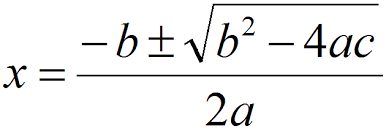f(x) = (x + 3)(x - 5)

Then x = -3 and x = 5 make f(x) zero.The above answer was easy because f(x) was factored. The answer is less obvious for

f(x) = x2 - 2x - 15

Of course, in general, the coefficients are not integers, which makes the problem even more difficult (unless you have a calculator):

g(x) = 4.592x2 + 2.308x - 8.083

Fortunately, the quadratic formula provides a way to find the roots. If the quadratic is

ax2 + bx + c

then the formula gives your the roots, if any.

QUESTION 2:

(Math Review:) Will there be two roots for every choice of a, b, and c?